Courses

Dynamical Systems (Part - 2) - Classical Mechanics, UGC - NET Physics Notes | Study Physics for IIT JAM, UGC - NET, CSIR NET - Physics

Physics: Dynamical Systems (Part - 2) - Classical Mechanics, UGC - NET Physics Notes | Study Physics for IIT JAM, UGC - NET, CSIR NET - Physics

The document Dynamical Systems (Part - 2) - Classical Mechanics, UGC - NET Physics Notes | Study Physics for IIT JAM, UGC - NET, CSIR NET - Physics is a part of the Physics Course Physics for IIT JAM, UGC - NET, CSIR NET.
All you need of Physics at this link: Physics

Critical points of the pendulum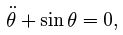or, in the form of dynamical system,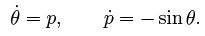(8.7)

What are possible equilibrium positions of the pendulum? Clearly, if we set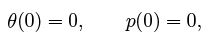the pendulum will not move. These conditions correspond to the situation when the pendulum is hanging freely at the equilibrium position with zero initial velocity. In this case the derivatives of θ and p take values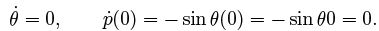In other words, the derivatives of all variables xa, where x = (θ,  p), vanish and therefore the pendulum does not move.
However, there is another possibility. If we set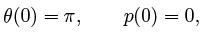then the derivatives will vanish as well: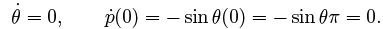This corresponds to situation when the pendulum is in upper position. If we were able to arrange initial conditions in such a way that the angle of de
ection θ is exactly equal to π and the velocity is zero, we would obtain an equilibrium con guration in which the pendulum does not move.

Points with these properties are called critical points or xed points. In general, critical point xC of dynamical system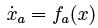is a point for which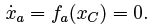If we choose the initial point to x(0) = xC , the system will remain at this initial position forever, it will not move. For mathematical pendulum we have two critical points,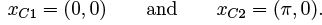They are sketched in gure 8.6.
On the other hand, we feel that two critical points of mathematical pendulum have a di
erent character. The rst critical point xC 1 is stable in the sense that small perturbation results in periodic oscillations near this critical point. Critical point xC 2 is unstable in the sense that arbitrarily small perturbation will cause the fall of pendulum and results in oscillations around critical point xC 1!

This observation is based on our physical intuition but can we predict stability or instability of critical points directly from equations? By de nition, critical points represent equilibrium con gurations of the system. Can we predict the behaviour of the system near the critical point?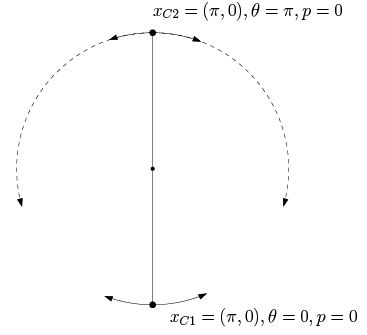Fig. 8.6. Two critical points of mathematical pendulum.

The idea is that small perturbations of stable critical points will produce small deviations from equilibrium position, but small perturbations of unstable critical points will result in a motion far from the critical point. We will use a basic fact from mathematical analysis that, under certain assumptions, function f (x) can be expanded into the Taylor series around arbitrary point xC in the following way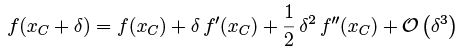(8.8)

where f'(x) denotes the value of derivative of f at point x, f"(x) is the second derivative at the x, etc.
First we analyse critical point xC 1 = (0; 0). Let us denote critical values of θ = x1 and p = x2 by

θC = 0, pC = 0

Now suppose that the angle θ is only a small perturbation of θC , i.e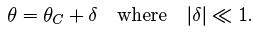Since θC = 0 is a constant, we have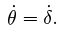Next we simplify equations of motion (8.7) under this assumption. Let us expand sin θ around critical point θC = 0: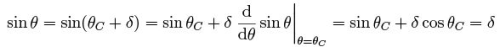where we have neglected higher powers of δ which is assumed to be small. With this assumption, equations of pendulum (8.7) simplify to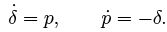These are, in fact, well-known equations for harmonic oscillator and we can easily plot the solution which we already know is a circle. We can plot it by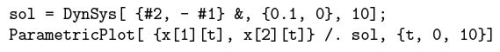where we have chosen small initial deflection θ(0) = 0.1, in accordance with the assumption. Since the solution is a circle, we observe that phase tra jectories near the rst critical point remain in the vicinity of this critical point; an indicator of stability.

Let us now investigate the second critical point located at

θC = π, pC = 0,

As in the previous case, we assume that deviations from critical value θC are small and write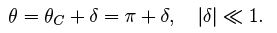Now we expand sin θ into the Taylor series about θ = θC as follows: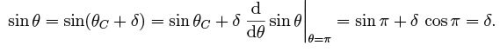Equations of motion (8.7) simplify to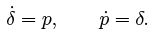In order to compare solutions near both critical points we use the following code: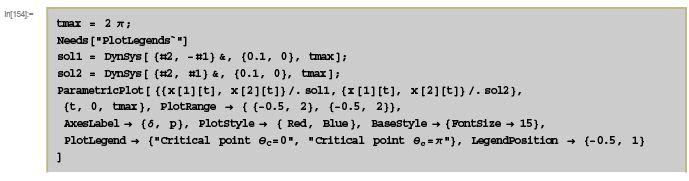Both trajectories near critical points are plotted in gure 8.7. We can see that trajectory corresponding to rst critical point θC = 0 is a circle and thus remains in the vicinity of the critical point. The second tra jectory corresponding to critical point θC = π, on the other hand, is a line which escapes to in nity. Hence, we can see that the second critical point is unstable in the following sense. If we move the pendulum to θ = π and set the initial velocity to zero, the pendulum remains at this equilibrium position. However, arbitrarily small perturbation (in our case δ = 0:1) will cause the pendulum to escape from equilibrium position quickly. In our case, the tra jectory escapes to in nity, but this is an artefact of the linearization: we have assumed that the perturbation δ is small but as soon as the pendulum is far enough from the critical point, this assumption is not valid anymore.

Stability of critical points

Having illustrated the main idea about the stability and instability on the example of mathematical pendulum, we can proceed to a general theory. For simplicity we restrict ourselves to autonomous planar dynamical systems, i.e. dynamical systems with only two variables x1 = x and x2 = y which can be visualised in the plane.
Hence, planar dynamical system is a set of two rst-order equations of the form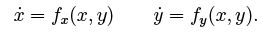(8.9)

Critical point of system (8.9) is such a point (xC , yC ) for which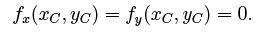(8.10)

Then the equations of motion (8.9) reduce to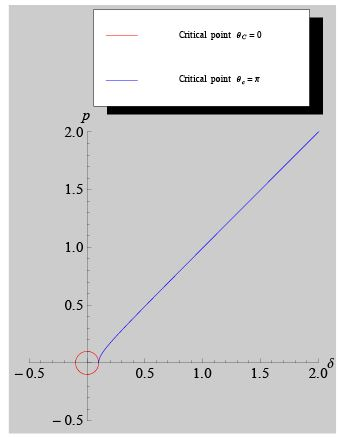Fig. 8.7. Phase tra jectories near two critical points θC = 0 and θC = π. In both cases the actual deflection is θ = θC + δ but with different θC . We can see that the red trajectory is a circle about the origin while the blue tra jectory diverges to in nity rapidly.

which means that critical points represent the equilibrium con gurations of the system.
Now we want to investigate the stability or instability of critical points. That means we want to nd out how the phase tra jectories behave in the vicinity of critical points. In the case of the pendulum we have seen that an appropriate way how to proceed is to linearize the system of equations near the critical point.
Let us assume that (xC, yC ) is a critical point of system (8.9). In the neighbourhood of critical point we can write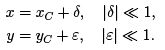(8.11)

Since xC and yC are constants, for the time derivatives of x and y we have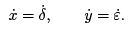Function fx(x, y) can be then expanded into the Taylor series: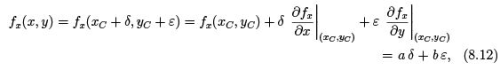where we have used de nition (8.10) in the last step and denoted partial derivatives of fx by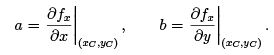(8.13)

Vertical line with the subscript indicates that partial derivatives must be evaluated at the critical point. Similarly, for fy we find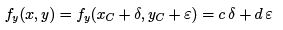where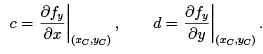(8.14)

Thus, near the critical point, planar dynamical system (8.9) can be replaced by simpler equations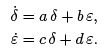(8.15)

Coefficients a; b; c and d are not functions but constants given by (8.13) and (8.14).
It is useful to write equations (8.15) in the matrix form. Let us de ne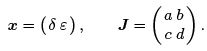Then two equations (8.15) are equivalent to single matrix equation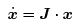(8.16)

where the dot denotes standard matrix multiplication.

Example

Let us consider non-linear dynamical system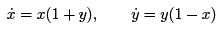with

fx = x(1 + y), fy = y(1 - x):

First we nd the critical points, i.e. we set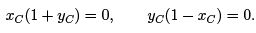There are two solution to these equations,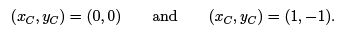We analyse these points separately. The emphasis is on nding the critical points and deriving linearized equations of motion. The solution is merely stated because we will analyse all cases in detail later.

a) Critical point (0, 0). In this case we write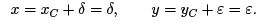Now we have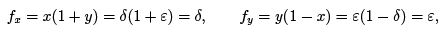where we have neglected higher order terms "∈δ because of linearization. Hence, in the neighbourhood of the rst critical point, the equations of motion are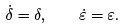These equations can be solved trivially to nd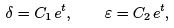where C1 and C2 are integration constants. We will discus this later, but for now it is obvious that the phase tra jectory escapes to in nity because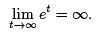Hence, this critical point is unstable.

b) Critical point (1, 1). In this case we write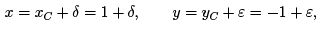so that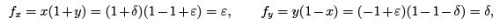where we have neglected products "∈δ again. Now the linearized equations of motion are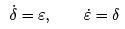which solve to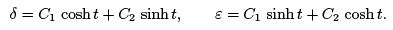The reader is invited to check that solutions (δ, ∈) are hyperbolas escaping to in nity and hence the second critical point is unstable again.

The document Dynamical Systems (Part - 2) - Classical Mechanics, UGC - NET Physics Notes | Study Physics for IIT JAM, UGC - NET, CSIR NET - Physics is a part of the Physics Course Physics for IIT JAM, UGC - NET, CSIR NET.
All you need of Physics at this link: PhysicsUse Code STAYHOME200 and get INR 200 additional OFF Use Coupon CodePhysics for IIT JAM, UGC - NET, CSIR NET

159 docs

Track your progress, build streaks, highlight & save important lessons and more!

,

,

,

,

,

,

,

,

,

,

,

,

,

,

,

,

,

,

,

,

,

,

,

,

,

,

,

,

,

,

;